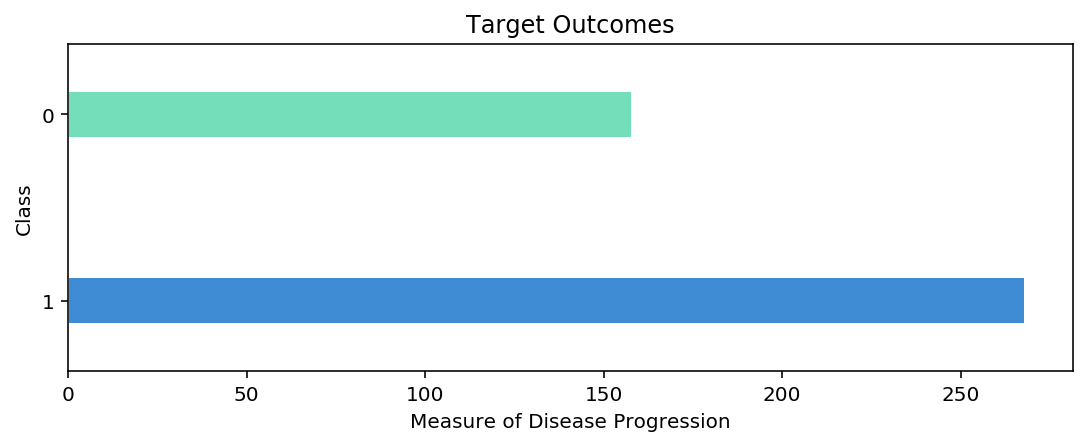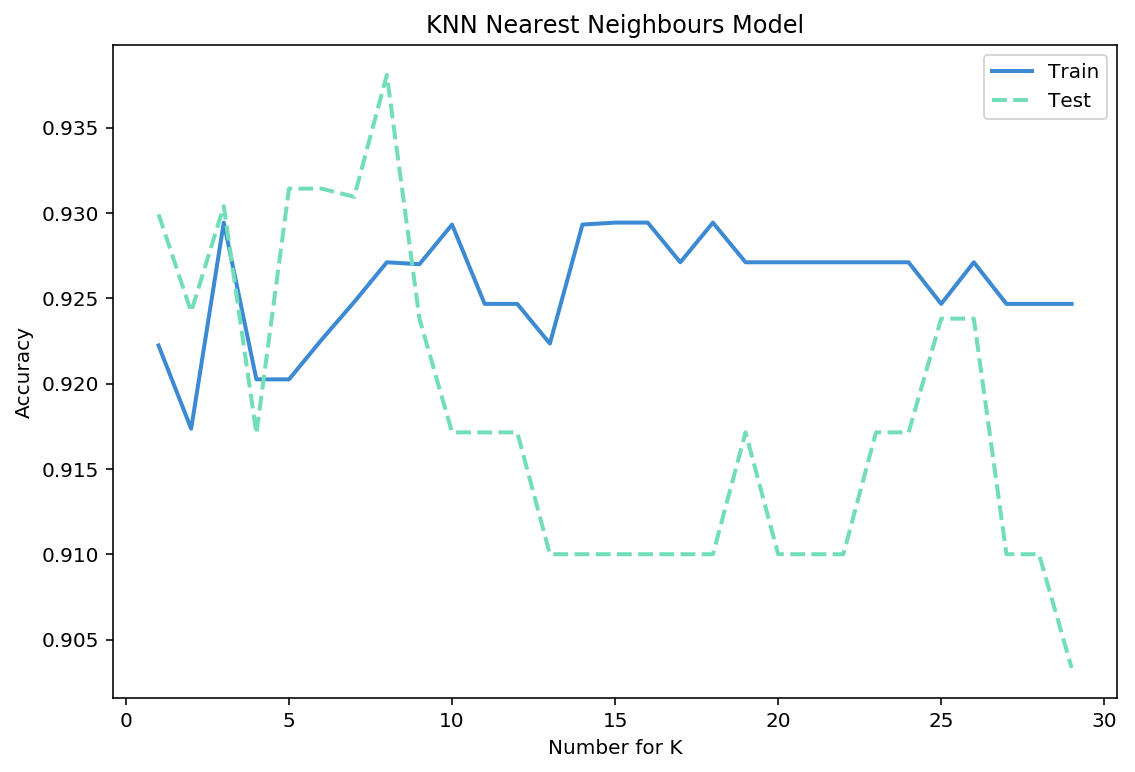01 Aug

## K-Nearest Neighbours Classifier

KNN is a very simple machine learning algorithim. Given a distance parameter and nearest neighbours parameter. The algorithim use premise that points that are related to one class are closely associated with each other. The algorithim will group each random data points place hyperplane using its K-Nearest Neighbours.

### Import Preliminaries¶

In :
%matplotlib inline
%config InlineBackend.figure_format='retina'

# Import modules
import matplotlib.pyplot as plt
import matplotlib as mpl
import numpy as np
import os
import pandas as pd
import sklearn
import seaborn as sns
import warnings

from sklearn.metrics import confusion_matrix
from sklearn.model_selection import train_test_split, cross_val_score
from sklearn.neighbors import KNeighborsClassifier

# Set pandas options
pd.set_option('max_columns',1000)
pd.set_option('max_rows',30)
pd.set_option('display.float_format', lambda x: '%.3f' % x)

# Set plotting options
mpl.rcParams['figure.figsize'] = (9.0, 3.0)

# Set warning options
warnings.filterwarnings('ignore');


### Import Data¶

In :
# Import data
X, y = breast_cancer.data, breast_cancer.target

# Train-test split the data
train_x, test_x, train_y, test_y = train_test_split(X,y)

# View the training dataframe

Out:
mean radius mean texture mean perimeter mean area mean smoothness mean compactness mean concavity mean concave points mean symmetry mean fractal dimension radius error texture error perimeter error area error smoothness error compactness error concavity error concave points error symmetry error fractal dimension error worst radius worst texture worst perimeter worst area worst smoothness worst compactness worst concavity worst concave points worst symmetry worst fractal dimension
0 11.360 17.570 72.490 399.800 0.089 0.053 0.028 0.021 0.160 0.059 0.192 1.555 1.359 13.660 0.005 0.010 0.012 0.006 0.013 0.002 13.050 36.320 85.070 521.300 0.145 0.162 0.181 0.087 0.297 0.077
1 15.780 22.910 105.700 782.600 0.116 0.175 0.213 0.095 0.210 0.073 0.552 1.072 3.598 58.630 0.009 0.040 0.059 0.014 0.015 0.006 20.190 30.500 130.300 1272.000 0.185 0.492 0.736 0.203 0.327 0.125
2 14.540 27.540 96.730 658.800 0.114 0.160 0.164 0.074 0.230 0.071 0.370 1.033 2.879 32.550 0.006 0.042 0.047 0.011 0.019 0.005 17.460 37.130 124.100 943.200 0.168 0.658 0.703 0.171 0.422 0.134
3 11.850 17.460 75.540 432.700 0.084 0.056 0.027 0.023 0.188 0.057 0.207 1.238 1.234 13.880 0.008 0.015 0.014 0.009 0.018 0.002 13.060 25.750 84.350 517.800 0.137 0.176 0.132 0.091 0.310 0.070
4 13.000 25.130 82.610 520.200 0.084 0.051 0.012 0.018 0.167 0.054 0.262 1.232 1.657 21.190 0.006 0.009 0.006 0.006 0.012 0.002 14.340 31.880 91.060 628.500 0.122 0.109 0.045 0.059 0.231 0.063

### Targets¶

In :
# Plot a barplot of the target clasees
pd.Series(train_y).value_counts().plot.barh(grid=False, color=['#3F8CD5','#73DEB9'], width=0.25,edgecolor='w')
plt.title('Target Outcomes')
plt.ylabel('Class')
plt.xlabel('Measure of Disease Progression');### Fit the Model¶

In :
# Fit the intial knn model
knn_model = KNeighborsClassifier(n_neighbors=5)
knn_model.fit(train_x, train_y);


### Model Evaluation¶

##### Cross Validation Score¶
In :
# View the cross validation score of the intial model
scores = cross_val_score(knn_model, train_x, train_y, cv=10,
scoring='accuracy')
print(f'Cross Validation Score: {scores.mean():.5f}')

Cross Validation Score: 0.92025

##### Confustion Matrix¶
In :
# Training Confusion Matrix
cmatrix = pd.DataFrame(confusion_matrix(train_y, knn_model.predict(train_x)))
cmatrix.index.name = 'class'
cmatrix['result'] = 'actual'
cmatrix.set_index('result', append=True, inplace=True)
cmatrix = cmatrix.reorder_levels(['result', 'class'])
cmatrix = cmatrix.stack()
cmatrix = pd.DataFrame(cmatrix)
cmatrix.columns = ['prediction']
cmatrix.unstack()

Out:
prediction
0 1
result class
actual 0 139 19
1 4 264
##### Parameter Tuning¶
In :
# Define paraameter range and score lists
neighbors_range = np.arange(1,30)
train_score = []
test_score = []

# Train a knn_model for every neighbour value in our list
for k in neighbors_range :
knn_model=KNeighborsClassifier(n_neighbors = k).fit(train_x,train_y)
train_score.append(cross_val_score(knn_model, train_x, train_y, cv=10, scoring='accuracy').mean())
test_score.append(cross_val_score(knn_model, test_x, test_y, cv=10, scoring='accuracy').mean())

# Plot our results
mpl.rcParams['figure.figsize'] = (9.0, 6.0)
plt.plot(neighbors_range,test_score,label="Test", linewidth=2,linestyle='--', color='#71DEB8')
plt.legend()
plt.title('KNN Nearest Neighbours Model')
plt.xlabel('Number for K')
plt.ylabel('Accuracy');### Final Model¶

In :
# Fit the final knn model
knn_model = KNeighborsClassifier(n_neighbors=3)
knn_model.fit(train_x, train_y);
scores = cross_val_score(knn_model, train_x, train_y, cv=10,
scoring='accuracy')
print(f'Cross Validation Score: {scores.mean():.5f}')

Cross Validation Score: 0.92944


### Predict Results¶

In :
# Viwe some predictions

Out:
0    1
1    1
2    0
3    1
4    0
5    1
6    1
dtype: int64

### General Notes¶

• Good baseline model
• The algorithm finds the closest points in the training dataset (it's neighbors).
• At minimum use 1 + number of your target classes in your dataset for your value of k
• The algorithm uses voting to assign the label, meaning it calculates the distance for itself and all other points. Then calculate its class by comparing its k - nearest data points. It assigns itself the class that is most frequent between its neighbors.
• The algorithm is computation intensive during the prediction portion of the model
• A small number of neighbor often work the best
• The algorithm often does not work well on data with high dimensionality and high sparsity (lots of zero)

Author: Kavi Sekhon